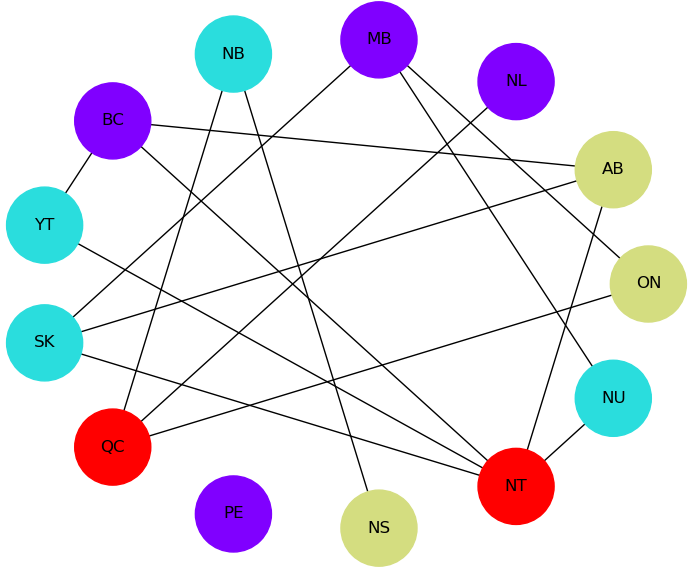# Map Coloring¶

This example solves a map-coloring problem to demonstrate using Ocean tools to solve a problem on a D-Wave system. It demonstrates using the D-Wave system to solve a more complex constraint satisfaction problem (CSP) than that solved in the example of Constrained Scheduling.

Constraint satisfaction problems require that all a problem’s variables be assigned values, out of a finite domain, that result in the satisfying of all constraints. The map-coloring CSP, for example, is to assign a color to each region of a map such that any two regions sharing a border have different colors.

The constraints for the map-coloring problem can be expressed as follows:

• Each region is assigned one color only, of $$C$$ possible colors.
• The color assigned to one region cannot be assigned to adjacent regions.

## Example Requirements¶

To run the code in this example, the following is required.

If you installed dwave-ocean-sdk and ran dwave config create, your installation should meet these requirements.

## Solution Steps¶

Following the standard solution process described in Section Solving Problems on a D-Wave System, we (1) formulate the problem as a binary quadratic model (BQM) by using unary encoding to represent the $$C$$ colors: each region is represented by $$C$$ variables, one for each possible color, which is set to value $$1$$ if selected, while the remaining $$C-1$$ variables are $$0$$. (2) Solve the BQM with a D-Wave system as the sampler.

The full workflow is as follows:

1. Formulate the problem as a graph, with provinces represented as nodes and shared borders as edges, using 4 binary variables (one per color) for each province.
2. Create a binary constraint satisfaction problem and add all the needed constraints.
3. Convert to a binary quadratic model.
4. Sample.
5. Plot a valid solution.

This example finds a solution to the map-coloring problem for a map of Canada using four colors (the sample code can easily be modified to change the number of colors or use different maps). Canada’s 13 provinces are denoted by postal codes:

Code Province Code Province
AB Alberta BC British Columbia
MB Manitoba NB New Brunswick
NL Newfoundland and Labrador NS Nova Scotia
NT Northwest Territories NU Nunavut
ON Ontario PE Prince Edward Island
YT Yukon

Note

You can skip directly to the complete code for the problem here: Map Coloring: Full Code.

The example uses the D-Wave binary CSP tool to set up constraints and convert the CSP to a binary quadratic model, dwave-system to set up a D-Wave system as the sampler, and NetworkX to plot results.

import dwavebinarycsp
from dwave.system.samplers import DWaveSampler
from dwave.system.composites import EmbeddingComposite
import networkx as nx
import matplotlib.pyplot as plt


Start by formulating the problem as a graph of the map with provinces as nodes and shared borders between provinces as edges (e.g., “(‘AB’, ‘BC’)” is an edge representing the shared border between British Columbia and Alberta).

# Represent the map as the nodes and edges of a graph
provinces = ['AB', 'BC', 'MB', 'NB', 'NL', 'NS', 'NT', 'NU', 'ON', 'PE',
'QC', 'SK', 'YT']
neighbors = [('AB', 'BC'), ('AB', 'NT'), ('AB', 'SK'), ('BC', 'NT'), ('BC', 'YT'),
('MB', 'NU'), ('MB', 'ON'), ('MB', 'SK'), ('NB', 'NS'), ('NB', 'QC'),
('NL', 'QC'), ('NT', 'NU'), ('NT', 'SK'), ('NT', 'YT'), ('ON', 'QC')]


Create a binary constraint satisfaction problem based on two types of constraints, where csp is the dwavebinarycsp CSP object:

• csp.add_constraint(one_color_configurations, variables) represents the constraint that each node (province) select a single color, as represented by valid configurations one_color_configurations = {(0, 0, 0, 1), (0, 0, 1, 0), (0, 1, 0, 0), (1, 0, 0, 0)}
• csp.add_constraint(not_both_1, variables) represents the constraint that two nodes (provinces) with a shared edge (border) not both select the same color.
# Function for the constraint that two nodes with a shared edge not both select
# one color
def not_both_1(v, u):
return not (v and u)

# Valid configurations for the constraint that each node select a single color
one_color_configurations = {(0, 0, 0, 1), (0, 0, 1, 0), (0, 1, 0, 0), (1, 0, 0, 0)}
colors = len(one_color_configurations)

# Create a binary constraint satisfaction problem
csp = dwavebinarycsp.ConstraintSatisfactionProblem(dwavebinarycsp.BINARY)

# Add constraint that each node (province) select a single color
for province in provinces:
variables = [province+str(i) for i in range(colors)]

# Add constraint that each pair of nodes with a shared edge not both select one color
for neighbor in neighbors:
v, u = neighbor
for i in range(colors):
variables = [v+str(i), u+str(i)]


Convert the CSP into a binary quadratic model so it can be solved on the D-Wave system.

bqm = dwavebinarycsp.stitch(csp)


The next code sets up a D-Wave system as the sampler and requests 50 samples.

Note

In the code below, replace sampler parameters as needed. If you configured a default solver, as described in Using a D-Wave System, you should be able to set the sampler without parameters as sampler = EmbeddingComposite(DWaveSampler()). You can see this information by running dwave config inspect in your terminal.

# Sample 50 times
sampler = EmbeddingComposite(DWaveSampler(endpoint='https://URL_to_my_D-Wave_system/', token='ABC-123456789012345678901234567890', solver='My_D-Wave_Solver'))


Note

The next code requires Matplotlib.

Plot a valid solution.

# Function that plots a returned sample
def plot_map(sample):
G = nx.Graph()
# Translate from binary to integer color representation
color_map = {}
for province in provinces:
for i in range(colors):
if sample[province+str(i)]:
color_map[province] = i
# Plot the sample with color-coded nodes
node_colors = [color_map.get(node) for node in G.nodes()]
nx.draw_circular(G, with_labels=True, node_color=node_colors, node_size=3000, cmap=plt.cm.rainbow)
plt.show()

# Plot the lowest-energy sample if it meets the constraints
sample = next(response.samples())
if not csp.check(sample):
print("Failed to color map")
else:
plot_map(sample)


The plot shows a solution returned by the D-Wave solver. No provinces sharing a border have the same color.Solution for a map of Canada with four colors. The graph comprises 13 nodes representing provinces connected by edges representing shared borders. No two nodes connected by an edge share a color.

Note

You can copy the complete code for the problem here: Map Coloring: Full Code.